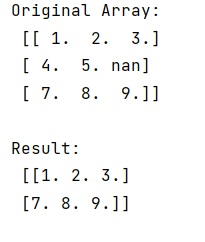# How to remove all rows in a numpy ndarray that contain non numeric values?

Given a NumPy ndarray, we have to remove all rows in a numpy ndarray that contain non numeric values.
Submitted by Pranit Sharma, on January 06, 2023

NumPy is an abbreviated form of Numerical Python. It is used for different types of scientific operations in python. Numpy is a vast library in python which is used for almost every kind of scientific or mathematical operation. It is itself an array which is a collection of various methods and functions for processing the arrays.

## Removing all rows in a numpy ndarray that contain non numeric values

Suppose that we are given with a ndarray with 3 rows out of which 1 row contains some non-numeric value and we need to remove this row from this numpy array.

For this purpose, we will use the isnan() method. The np.isnan(arr) returns a similar array with True where NaN, False elsewhere.

Along with this method, we will use any(axis=1) method which reduces an m*n array to n with an logical or operation on the whole rows, ~ (tilde sign) inverts True/False and arr[] chooses just the rows from the original array, which have True within the brackets.

Let us understand with the help of an example,

## Python code to remove all rows in a numpy ndarray that contain non numeric values

```# Import numpy
import numpy as np

# Creating an array
arr = np.array([[1,2,3], [4,5,np.nan], [7,8,9]])

# Display original array
print("Original Array:\n",arr,"\n")

# Removing rows having nan value
res = arr[~np.isnan(arr).any(axis=1)]

# Display result
print("Result:\n",res,"\n")
```

Output: